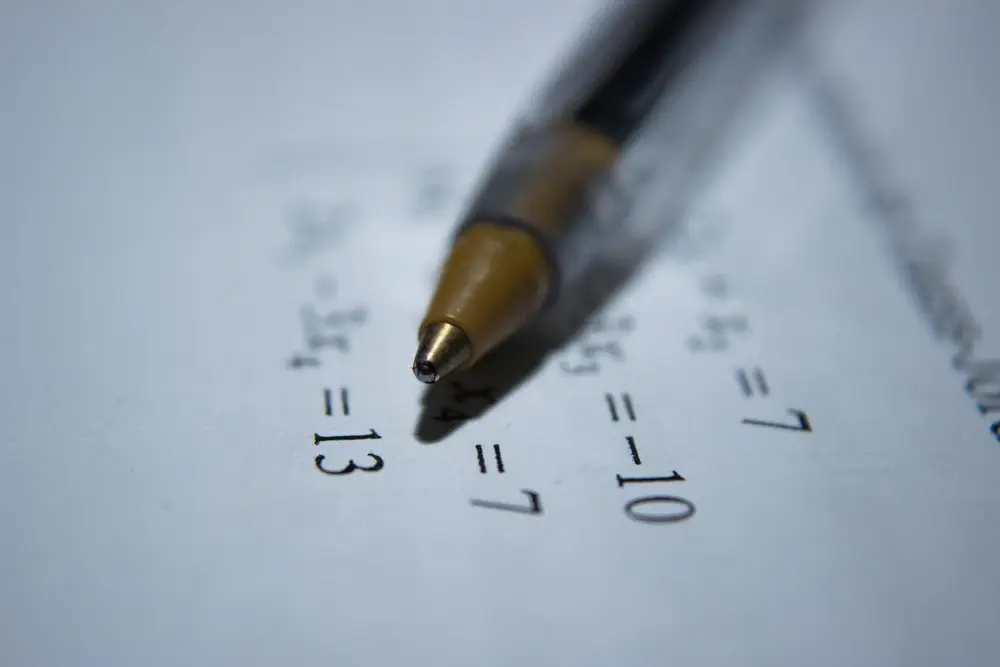# Algebraic equations

• Your maths exam will definitely have something which involves algebra. You will need to understand how to use and manipulate algebraic expressions to answer these questions.
• There are many rules in using algebra:
• An algebraic expression involves letters which represent values. There is an equals sign which shows you what the subject of the expression is equal to.
• To rearrange or solve an expression you may need to cancel things out. This may involve a calculation to one side of the expression. However, what you do to one side of the expression you must do the same to the other side.
• Before doing anything you should simplify an expression so that there are no fractions and no brackets.
• Multiplying out brackets is important. When multiplying out two brackets you must remember that everything has to be multiplied together:
• (a+b)(x+y)=ax+ay+bx+by
Algebra

Algebra

Algebra

Algebra

Algebra

Algebra